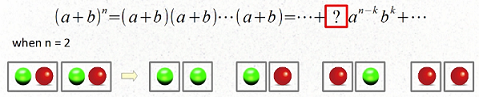The Binomial Theorem Intermediate

Video tutorial

Lecture Notes

The binomial theorem, also referred as binomail expansion: $$(a+b)^n = C_n^0a^n+C_n^1a^{n-1}b+\cdots + C_n^{n-1}ab^{n-1} + C_n^nb^n$$

It is helpful to understand its counting representation. One application of such understanding is to generalize this binomial theorem to the multinomial theorem which will be discussed later.